EnchantedLearning.com is a user-supported site.
As a bonus, site members have access to a banner-ad-free version of the site, with print-friendly pages.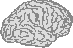More Math EnchantedLearning.comPath-o-MathTM PuzzlesLogic Puzzles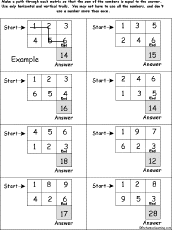Addition Path-o-Math Puzzles: 2 by 3Make a path through each number matrix so that the sum of the numbers is equal to the given answer. Use only horizontal and vertical trails. You may not have to use all the numbers, and don't use a number more than once. Puzzle #1, or the answers Puzzle #2, or the answers Puzzle #3, or the answers Puzzle #4, or the answers Puzzle #5, or the answers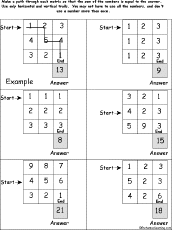Addition Path-o-Math Puzzles: 3 by 3Make a path through each number matrix so that the sum of the numbers is equal to the given answer. Use only horizontal and vertical trails. You may not have to use all the numbers, and don't use a number more than once. Puzzle #1, or the answers Puzzle #2, or the answers Puzzle #3, or the answers Puzzle #4, or the answers Puzzle #5, or the answers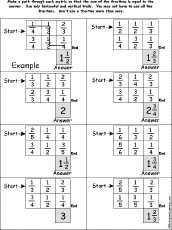Adding Fractions Path-o-Math Puzzles: 2 by 3Make a path through each number matrix so that the sum of the fractions is equal to the given answer. Use only horizontal and vertical trails. You may not have to use all the numbers, and don't use a number more than once. Puzzle #1, or the answers Puzzle #2, or the answers Puzzle #3, or the answers Puzzle #4, or the answers Puzzle #5, or the answers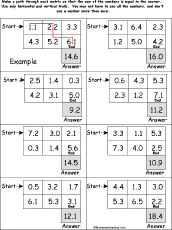Adding Decimals Path-o-Math Puzzles: 2 by 3Make a path through each number matrix so that the sum of the decimals is equal to the given answer. Use only horizontal and vertical trails. You may not have to use all the numbers, and don't use a number more than once. Puzzle #1, or the answers Puzzle #2, or the answers Puzzle #3, or the answers Puzzle #4, or the answers Puzzle #5, or the answers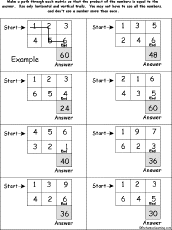Multiplying Numbers Path-o-Math Puzzles: 2 by 3Make a path through each number matrix so that the product of the numbers is equal to the given answer. Use only horizontal and vertical trails. You may not have to use all the numbers, and don't use a number more than once. Puzzle #1, or the answers Puzzle #2, or the answers Puzzle #3, or the answers Puzzle #4, or the answers Puzzle #5, or the answers

 +, - EnchantedLearning.comMath x, ÷
 A B C D E F G H I J K L M N O P Q R S T U V W X Y Z
 Number Line Fractions Decimals Measurement Rounding Graphs

Enchanted Learning®
Over 35,000 Web Pages
Sample Pages for Prospective Subscribers, or click below

 Overview of Site What's New Enchanted Learning Home Monthly Activity Calendar Books to Print Site Index K-3 Crafts K-3 Themes Little ExplorersPicture dictionary PreK/K Activities Rebus Rhymes Stories Writing Cloze Activities Essay Topics Newspaper Writing Activities Parts of Speech Fiction The Test of Time Biology Animal Printouts Biology Label Printouts Biomes Birds Butterflies Dinosaurs Food Chain Human Anatomy Mammals Plants Rainforests Sharks Whales Physical Sciences: K-12 Astronomy The Earth Geology Hurricanes Landforms Oceans Tsunami Volcano Languages Dutch French German Italian Japanese (Romaji) Portuguese Spanish Swedish Geography/History Explorers Flags Geography Inventors US History Other Topics Art and Artists Calendars College Finder Crafts Graphic Organizers Label Me! Printouts Math Music Word Wheels

## Enchanted Learning Search

 Search the Enchanted Learning website for: